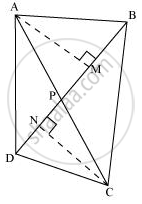# Diagonals AC and BD of a quadrilateral ABCD intersect each other at P. Show that ar (APB) × ar (CPD) = ar (APD) × ar (BPC). - Mathematics

Diagonals AC and BD of a quadrilateral ABCD intersect each other at P. Show that ar (APB) × ar (CPD) = ar (APD) × ar (BPC).

[Hint : From A and C, draw perpendiculars to BD.]

#### Solution

Let us draw AM ⊥ BD and CN ⊥ BD"Area of a triangle "=1/2xx"Base"xx"Altitude"

ar(APB)xxar(CPD)=[1/2xxBPxxAM]xx[1/2xxPDxxCN]

=1/4xxBPxxAMxxPDxxCN

ar(APD)xxar(BPC)=[1/2xxPDxxAM]xx[1/2xxCNxxBP]

=1/4xxPDxxAMxxCNxxBP

=1/4xxBPxxAMxxPDxxCN

∴ ar (APB) × ar (CPD) = ar (APD) × ar (BPC)

Concept: Corollary: Triangles on the same base and between the same parallels are equal in area.
Is there an error in this question or solution?

#### APPEARS IN

NCERT Class 9 Maths
Chapter 9 Areas of Parallelograms and Triangles
Exercise 9.4 | Q 6 | Page 166